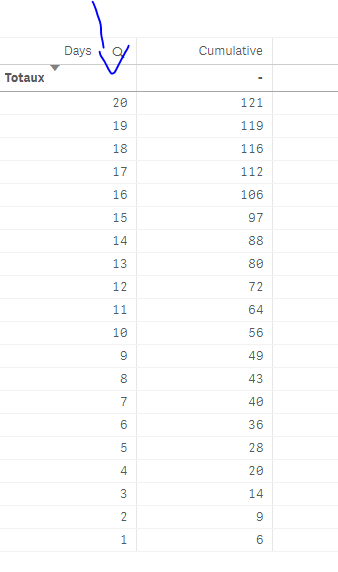New to Qlik Sense

Discussion board where members can get started with Qlik Sense.

Announcements
QlikWorld 2020: Join us May 11 - 14, 2020 in Phoenix, AZ. Register early and save \$400. Learn More
Highlighted
Contributor

cumulative table with sum cash applied versus total invoiced\$ per month

Dear all,

I have table with the following dimension and measurement:

Dimension:

day([Month date])

Measurement:

(Sum({<[Year Month]={'\$(Current_Month_YYYYMM)'}>}[Cash App Amt USD])

/

Sum({<[Year Month]={'\$(Current_Month_YYYYMM)'},[Trans Type]={'invoice'}>}total <[Year Month]>[Trans Amt USD]))

Table now shows:

 Days Measurement 1 6 2 3 3 5 4 6 5 8 6 8 7 4 8 3 9 6 10 7 11 8 12 8 13 8 14 8 15 9 16 9 17 6 18 4 19 3 20 2

Now I want to see this result as cumulative.

 Day Cumulative 1 6 2 9 3 14 4 20 5 28 6 36 7 40 8 43 9 49 10 56 11 64 12 72 13 80 14 88 15 97 16 106 17 112 18 116 19 119 20 121

How do I need to do this?

Ugur

Tags (3)
9 RepliesPartner

Re: cumulative table with sum cash applied versus total invoiced\$ per month

from:

sum(YourMeasure)

do :

aggr(

RangeSum(above(

Sum({<Days>}Measurement)

,0,RowNo()))

,(Days,ascend))

Result:Even iuf u select a day, u'll have the cumulative sum well calculated:and with the aggr() part, even if ur table is not sorted by ascending days, it'll still be calculated well:Contributor

Re: cumulative table with sum cash applied versus total invoiced\$ per month

aggr(

RangeSum(above(

Sum({<Days>}

[Year Month]={'\$(Current_Month_YYYYMM)'}>}[Cash App Amt USD])

/

Sum({<[Year Month]={'\$(Current_Month_YYYYMM)'},[Trans Type]={'invoice'}>}total <[Year Month]>[Trans Amt USD])

)

,0,RowNo()))

,(Days,ascend))

This didn't work...

Contributor

Re: cumulative table with sum cash applied versus total invoiced\$ per month

aggr(

RangeSum(

above(

Sum({<[Month date]>}

[Year Month]={'\$(Current_Month_YYYYMM)'}>}[Cash App Amt USD])

/

Sum({<[Year Month]={'\$(Current_Month_YYYYMM)'},[Trans Type]={'invoice'}>}total <[Year Month]>[Trans Amt USD])

)

,0

,RowNo()))

,([Month date],ascend))

Contributor

Re: cumulative table with sum cash applied versus total invoiced\$ per month

didn't work alsoPartner

Re: cumulative table with sum cash applied versus total invoiced\$ per month

aggr(

RangeSum(above(

(Sum({<Days, [Year Month]={'\$(Current_Month_YYYYMM)'}>}[Cash App Amt USD])

/

Sum({<Days,[Year Month]={'\$(Current_Month_YYYYMM)'},[Trans Type]={'invoice'}>}total <[Year Month]>[Trans Amt USD]))

,0,RowNo()))

,(Days,ascend)

Contributor

Re: cumulative table with sum cash applied versus total invoiced\$ per month

Hi Omar, I couldn't define days. the days field is extracted from a formula to extract out of a day with using day([Month Date]). Can you please sent me the printscreens of the formula you put in your qliksense file.

Thanks

Ugur

Contributor

Re: cumulative table with sum cash applied versus total invoiced\$ per month

I appreciate your help very very much, thanks for learning me.

Contributor

Re: cumulative table with sum cash applied versus total invoiced\$ per month

Omar are you able to help me please?Partner

Re: cumulative table with sum cash applied versus total invoiced\$ per month

Can't u create the days dimension in the script then us it?